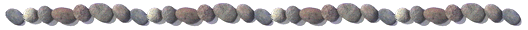Mathematica package BVPh 2.0

PublicationsThe HAM-based Mathematica package BVPh 1.0 can only deal with BVPs of single ordinary differential equation (ODE); it cannot solve systems of ODEs. Now the newest version BVPh 2.0, can deal with many systems of coupled ordinary differential equations (ODEs) defined in finite and/or semi-infinite intervals. Besides, new algorithms are used in some modules of BVPh 2.0. Hence, BVPh 2.0 is much faster than BVPh 1.0 in most cases.  For more detail about BVPh 2.0, please refer to here [PDF].

The package BVPh 2.0 is developed on Mathematica 7.0. As some new features of Mathematica 7.0 are used, we strongly recommend you to use the BVPh 2.0 in Mathematica 7.0 or higher version, or it will not work.

Input Data Files of Examples:

Illustrative Example:

Example 1:

Example 2:

Example 2

Example 3:

Example 3

Example 4:

Example 4

Example 5: